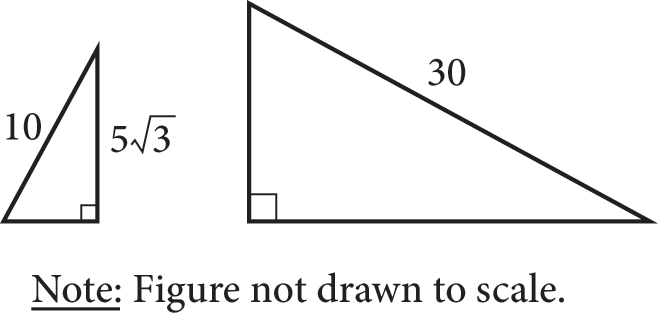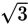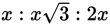# SAT Math Multiple Choice Question 965: Answer and Explanation

### Test Information

Question: 965

1.If the right triangles in the figure shown are similar triangles, what is the lengthof the shorter leg of the larger triangle?

• A. 10
• B. 15
• C. 10• D. 15Strategic Advice: Whenever you seein a triangle problem, think about special right triangles. Don't forget that the ratios of the sides of these triangles are given on the reference page at the beginning of each math section.
Getting to the Answer: Examine the smaller triangle: When the sides of a right triangle are in the ratio, the triangle is a 30-60-90 triangle. Knowing that two of the sides of the smaller triangle fit this ratio is enough information to draw the conclusion that it is a 30-60-90 triangle (although you could use the Pythagorean theorem to confirm that the shorter leg has length 5 if you're not convinced). Because the two triangles are similar, you know that their angle measures are equal, and the larger triangle is also a 30-60-90 triangle. In the ratio, 2x represents the length of the hypotenuse and x represents the length of the shorter leg. According to the figure, the hypotenuse of the larger triangle has length 30, so solve 2x = 30 to find that the length of the shorter leg of the larger triangle has length x = 15, which is (B).